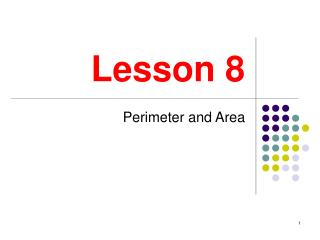DownloadDownload PresentationLesson 8

# Lesson 8

Télécharger la présentation## Lesson 8

- - - - - - - - - - - - - - - - - - - - - - - - - - - E N D - - - - - - - - - - - - - - - - - - - - - - - - - - -
##### Presentation Transcript

1. Lesson 8 Perimeter and Area

2. Perimeter • The perimeter of a closed figure is the distance around the outside of the figure. • In the case of a polygon, the perimeter is found by adding the lengths of all of its sides. No special formulas are needed. • Units for perimeter include inches, centimeters, miles, etc.

3. C D A B Example • In the figure, ABCD is a rectangle, and What is the perimeter of this rectangle? • The opposite sides of a rectangle are congruent. So, • So, the perimeter is

4. Circumference • The distance around the outside of a circle is traditionally called the circumference of the circle, not the perimeter. • The circumference of a circle with diameter d is given by the formula • Here, (pi) is a mathematical constant equal to approximately

5. Example • The radius of a circle is 5 inches. • What is the circumference of this circle? Round to the nearest hundredth of an inch. • First, note that • So, • Note: scientific calculators have a button.

6. Example • In the figure, a rectangle is surmounted by a semicircle. • Given the measurements as marked, find the perimeter of the figure. • Note that the diameter of the circle is 10. So, the top and bottom sides of the rectangle are also 10. • The left side of the rectangle is 15. • The circumference of the semicircle is • So, the perimeter of the figure is 5 10 15 15 10

7. Area • The area of a closed figure (like a circle or a polygon) measures the amount of “space” the figure takes up. • For example, to find out how much carpet to order for a room, you would need to know the area of the room’s floor. • Units used for area include square centimeters square miles, square yards, and acres.

8. Area of a Rectangle • The area of a rectangle is found by multiplying its base times its height (or length times width). • If the base is b and the height is h as in the figure, then the area formula is • Note that the product of two length units gives area units (like: inches times inches equals square inches). h b

9. 10 Example • The figure shown is a square whose diagonal measures 10. • What is the area of the square? • Using our knowledge of 45-45-90 triangles, note that each side of the square must be • Now, since a square is a rectangle, we find its area by multiplying base times height:

10. h h h Altitudes of Triangles • The formula for the area of a triangle involves the length of an altitude of the triangle. So, first we discuss what an altitude is. • An altitude is a line segment that runs from one vertex of the triangle to the opposite side or extension of the opposite side, and it is perpendicular to this opposite side (or extension). • Some altitudes are drawn below and marked h:

11. Area of a Triangle • The formula for the area of a triangle is where b is the length of the base (one of the sides of the triangle) and h is the height (the length of the altitude drawn to the base).

12. A C 15 25 B Example • The triangle in the figure is a right triangle with right angle at A, and sides as marked. • Find the area of this triangle. • We will take AB as the base. Then the height would be AC, which we can find with the Pythagorean Theorem: • So, the area is: 20

13. h b Area of a Parallelogram • To find the area of a parallelogram multiply its base times its height. • The base is any side of the parallelogram like the one marked b in the figure. • The height is the length of an altitude drawn to the base like the one marked h in the figure.

14. b h B Area of a Trapezoid • To find the area of a trapezoid multiply the height by the mean of the two bases. • If the height is h and the bases are b and B as in the figure, then the area formula is:

15. Area of a Circle • The area of a circle with radius r is found by multiplying pi by the radius squared. • The formula is

16. Heron’s Formula • Heron’s Formula is used to find the area of a triangle when altitudes are unknown, but all three sides are known. • If the lengths of the sides of the triangle are a, b, and c, then the area is given by the formula where s is the semiperimeter: b a c

17. Adding Areas • If you have to find the area of a complex shape, try dissecting the shape into non-overlapping simple shapes that you can find the area of. Then add the areas of the simple shapes. • For example, note how the shape below is dissected into two rectangles.

18. Subtracting Areas • Sometimes the area of a complex figure, especially one with “holes” in it, can be found by subtracting the areas of simpler figures. • For example, to find the shaded area below, we would subtract the area of the circle from the area of the rectangle.